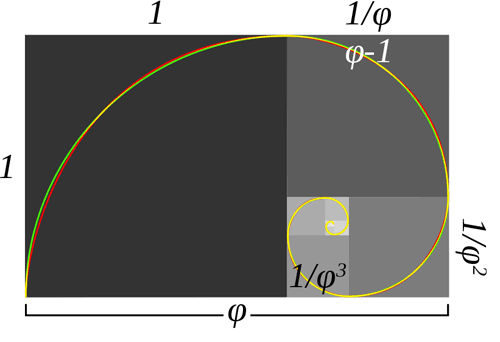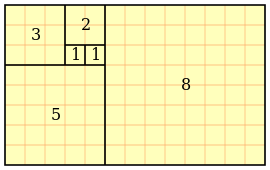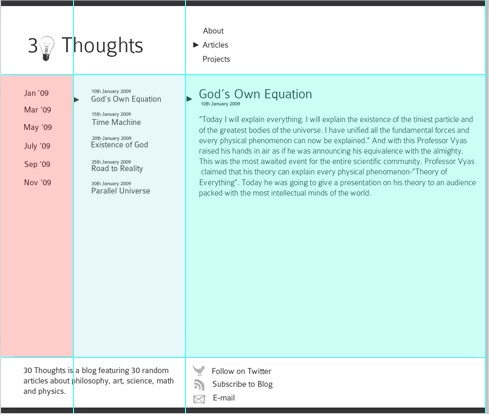# Fibonacci design

The first two Fibonacci numbers are 0 and 1, and each remaining number is the sum of the previous two.
The Fibonacci sequence is the sequence of integers: 0, 1, 1, 2, 3, 5, 8, 13, 21, 34, 55, 89, 144, 233, 377, 610, 987, 1597, 2584, ...
The higher the Fibonacci sequence gets, the closer its numbers relate to each other according to the Golden Ratio.
Fibonacci designs are designs based on the Fibonacci sequence of numbers.You can see that the page is divided into three columns. Each column corresponds to a Fibonacci number. For this design, we used a base width of 90 pixels. This base width is then multiplied by a Fibonacci number to get the total width for a particular column. For example, the first column has a width of 180 pixels (90 x 2); the second column has a width of 270 pixels (90 x 3); and the third column has a width of 720 pixels (90 x 8). The font size also corresponds to a Fibonacci number. The blog heading has a size of 55px; the article’s heading is 34px; and the content is 21px.